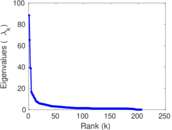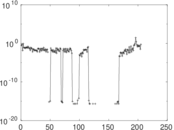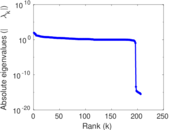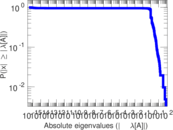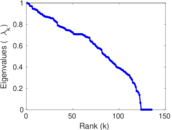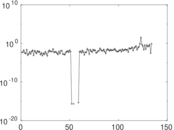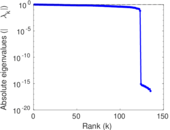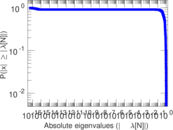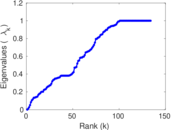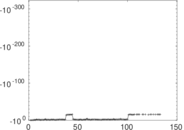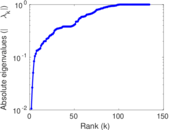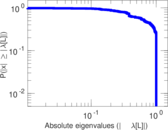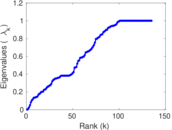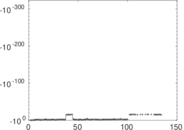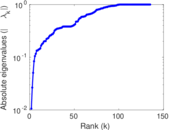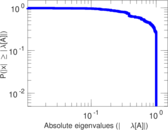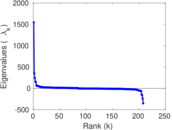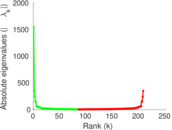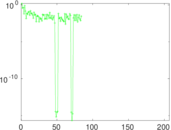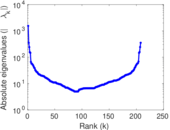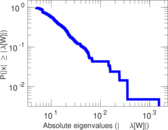# Wikiquote edits (cy)

This is the bipartite edit network of the Welsh Wikiquote. It contains users and pages from the Welsh Wikiquote, connected by edit events. Each edge represents an edit. The dataset includes the timestamp of each edit.

 Code `qcy` Internal name `edit-cywikiquote` Name Wikiquote edits (cy) Data source http://dumps.wikimedia.org/ AvailabilityDataset is available for download Consistency checkDataset passed all tests Category Authorship network Dataset timestamp 2017-10-20 Node meaning User, article Edge meaning Edit Network formatBipartite, undirected Edge typeUnweighted, multiple edges Temporal dataEdges are annotated with timestamps

## Statistics

 Size n = 1,557 Left size n1 = 208 Right size n2 = 1,349 Volume m = 3,591 Unique edge count m̿ = 2,492 Wedge count s = 263,535 Claw count z = 30,882,623 Cross count x = 3,100,979,019 Square count q = 79,443 4-Tour count T4 = 1,694,800 Maximum degree dmax = 849 Maximum left degree d1max = 849 Maximum right degree d2max = 143 Average degree d = 4.612 72 Average left degree d1 = 17.264 4 Average right degree d2 = 2.661 97 Fill p = 0.008 881 22 Average edge multiplicity m̃ = 1.441 01 Size of LCC N = 1,277 Diameter δ = 16 50-Percentile effective diameter δ0.5 = 3.635 33 90-Percentile effective diameter δ0.9 = 6.840 23 Median distance δM = 4 Mean distance δm = 4.455 30 Gini coefficient G = 0.693 265 Balanced inequality ratio P = 0.233 222 Left balanced inequality ratio P1 = 0.127 820 Right balanced inequality ratio P2 = 0.316 625 Relative edge distribution entropy Her = 0.797 198 Power law exponent γ = 2.866 20 Tail power law exponent γt = 2.481 00 Tail power law exponent with p γ3 = 2.481 00 p-value p = 0.000 00 Left tail power law exponent with p γ3,1 = 1.751 00 Left p-value p1 = 0.202 000 Right tail power law exponent with p γ3,2 = 6.311 00 Right p-value p2 = 0.872 000 Degree assortativity ρ = −0.085 092 6 Degree assortativity p-value pρ = 2.105 45 × 10−5 Spectral norm α = 88.453 3 Algebraic connectivity a = 0.010 559 4 Spectral separation |λ1[A] / λ2[A]| = 1.355 17 Controllability C = 1,078 Relative controllability Cr = 0.733 333

## Plots

### Fruchterman–Reingold graph drawing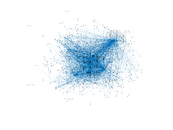### Degree distribution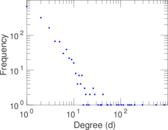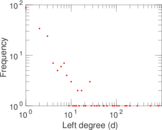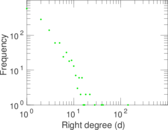### Cumulative degree distribution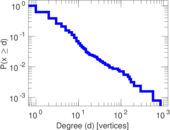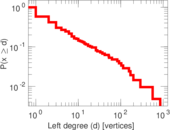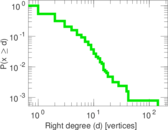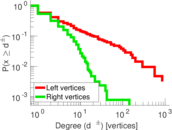### Lorenz curve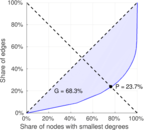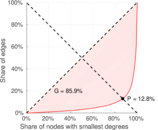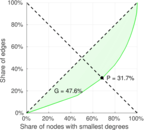### Spectral distribution of the adjacency matrix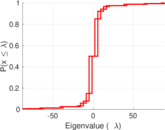### Spectral distribution of the normalized adjacency matrix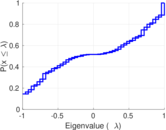### Spectral distribution of the Laplacian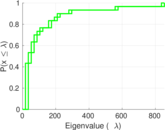### Spectral graph drawing based on the adjacency matrix### Spectral graph drawing based on the Laplacian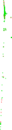### Spectral graph drawing based on the normalized adjacency matrix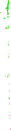### Degree assortativity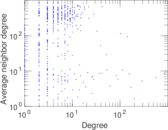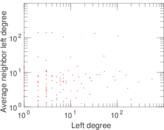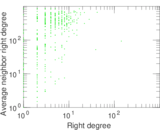### Zipf plot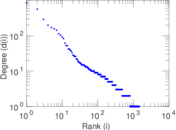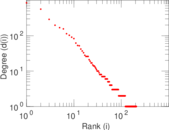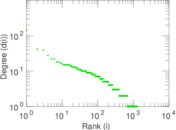### Hop distribution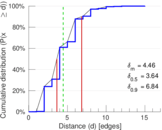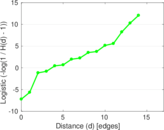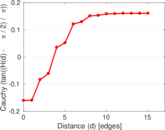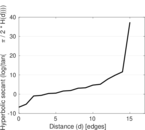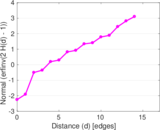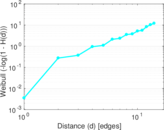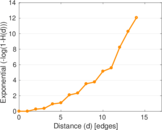### Double Laplacian graph drawing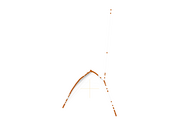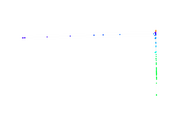### Delaunay graph drawing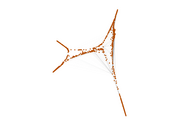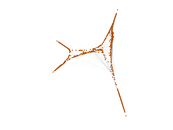### Edge weight/multiplicity distribution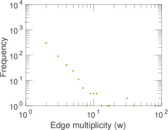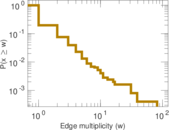### Temporal distribution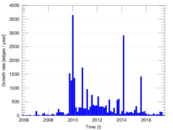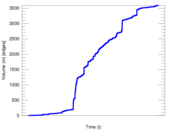### Temporal hop distribution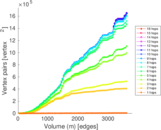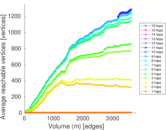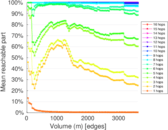### Diameter/density evolution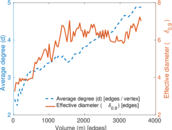### Matrix decompositions plots